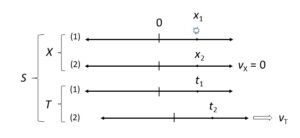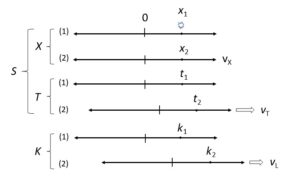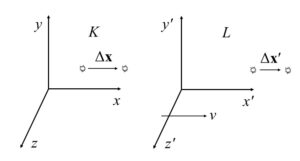iSoul In the beginning is reality.

# A theory of time

Note: as the research develops this post will be updated.

Speed is the travel distance per unit of travel time. In racing there is a measure of the travel time per unit of travel distance, which is called the pace. These are not exactly inverses since the denominated in different units. Note that a faster motion is indicated by a lower pace since it takes a shorter time to travel the same distance.

Velocity is a vector quantity whose magnitude is a body’s speed and whose direction is the body’s direction of motion. What is the opposite concept, a vector quantity whose magnitude is a body’s pace and whose direction is the body’s direction of motion? The dictionary lacks a word for this concept; I propose calling it lenticity [lentitude] from Latin lentus, slow, since a larger value indicates a slower motion.

Motion is a form of change, and change is characterized by difference. A body at rest does not change. A body in motion changes. But a body is at rest only with respect to another body at rest; they change the same way. A body is in motion only with respect to another body in motion; they change in different ways.

What is a body but something physical with some consistency; some attribute must not change. If something changes completely, it is not a body, or at least not a single body. What is the length of a body? It is the difference between one end and the other end. This difference is a change, a motion, with respect to the body or with respect to an observer at rest with respect to the body.

Since a body at rest is not moving, only one representation is required. But a body in motion requires two or more representations. Uniform motion requires a minimum of two representations. Accelerated motion requires a minimum of three representations. The order of representations indicates the temporal direction of motion.

An observer of an analogue clock is at rest relative to the clock dial but not the clock hands, yet the hands indicate the time for the observer. That is, there is the frame of reference for the clock dial and another frame of reference for the clock hands. This requirement for two frames of reference to measure time motives the following definitions.

An inertial reference frame (“frame”) is a physical system relative to which the position and distance of physical bodies can be measured. Frames have scales marked on them indicating coordinate positions. These frames do not have clocks. Frames are associated with observers, who are persons or devices that make measurements relative to their frames. For convenience, consider linear motion along one axis.

A rest frame of observer S is any frame, A, at rest relative to S. A motion frame of observer S is any frame, B, not at rest relative to S. Select a particular pair of rest frame and motion frame as the standard rate of motion, speed vT or pace wT. Other frame pairs with the same rate of motion are determined by comparison: frame pair R1 and M1 have the same relative speed  as frame pair R2 and M2 iff ΔR1/ΔM1 = ΔR2/ΔM2 = vT; and frame pair M1 and R1 have the same relative pace  as frame pair M2 and R2 iff ΔM1/ΔR1 = ΔM2/ΔR2 = wT.

Let two frames be associated with each observer, S: frames X and T so that every position has coordinates (x; t) for three-vectors x and t in frames X and T, respectively. The two frames are (1) a particular rest frame, X; and (2) a particular motion frame, T, (2a) moving with speed |vT| relative to X, such that |vT| = |Δxt| for any coordinates of event E (xi; ti), or (2b) moving with pace |wT| relative to X, such that |wT| = |Δtx| for any coordinates of event E (xi; ti).Given the above diagram, define Δx = x2x1 = 0 and Δt = t2t1. Then vx = Δx / Δt = 0 and vt = Δx′ / Δt′. In this way relative velocities are defined by frames without need for a clock.

Event E is at rest relative to frame X. Frame L is moving with velocity vL relative to frame K. Frame T is moving with velocity vT relative to frame X and |vT| is the standard speed of comparison. All speeds are determined by the relative positions of events and frames.An equation of motion is an equation of the coordinates f(x; t). The position of an event E relative to K is the location of E relative to K, i.e., its distance coordinates. The position of an event E relative to L is the chronation of E relative to L, i.e., its time (distime) coordinates.

A rest frame and a comparison frame are associated with each observer. In (3+1) only one dimension of an observer’s comparison frame is used, so the other two dimensions are hidden. In (1+3) only one dimension of an observer’s rest frame is used, so the other two dimensions are hidden.Lengths in one frame are related to other frames by their relative velocity: x′ = xvt, whereas durations are universal. Alternately, durations in one frame are related to other frames by their relative lenticity: t′ = twx, whereas lengths are universal.

Positions (chronations) on the dimension in relative motion of a comparison frame provide the time for the observer. That time is assigned to the observer’s whole rest frame for (3+1) coordinates. Positions (locations) on the dimension of the rest frame in motion relative to the comparison frame provide the stance for the observer. That stance is assigned to the observer’s who motion frame for (1+3) coordinates.

Let frame K with axes x1, y1, and z1 be a rest frame of observer P. Let frame L with axes x2 y2 and z2 be a motion frame of observer P. Let frame H with axes t, s, and r be a comparison frame of observer P with the frame H having the standard speed relative to K of vt and a standard pace relative to K of wt.

Simultaneous events coincide: they have the same position in either space or time.The position of a body relative to a frame, K, at rest relative to observer P, is proportional to its position in space relative to K. If frame, L, has the clock rate of motion relative to the frame K, then the position of an event, E, relative to K that is at rest relative to L is proportional to its position in time relative to K.

In order to ensure that a frame, L, is moving at a constant rate relative to frame K, take a body, P, at rest relative to the frame, K, and a body, Q, at rest relative to the frame L. If for any two events E1 and E2 such that

||(q2q1)|| = vt ||(p2p1)|| ≠ 0,

where p1 and p2 are the position vectors relative to K of E1 and E2, respectively, and q1 and q2 are the position vectors relative to L of E1 and E2, respectively, then vt is a constant. The change in position relative to L between events E1 and E2 is proportional to the change in time, and the change in position of relative to K between events E1 and E2 is proportional to the change in space (i.e., spatial location).

An observer P measures space coordinates relative to a frame K at rest relative to P. Call frame K a space frame for P. Space (3D space) is the R3 geometry of places and lengths in a space frame. A place point is a point in 3D space. The space origin is a reference place point. The location vector of a place point is the 3D space vector to it from the space origin. The coordinates of place points are called locations relative to the frame K of observer P and are measured in terms of length of space. Let the space axes in K be designated as x1, x2, and x3.

An observer P measures time coordinates relative to a frame L in uniform motion at a standard rate relative to P. Call frame L a time frame for P. Time (3D time) is the R3 geometry of times and durations in a time frame. A time point is a point in 3D time. The time origin is a reference time point. The chronation vector of a time point is the 3D time vector to it from the time origin. The coordinates of time points are called chronations relative to the frame L of observer P and are measured in terms of length of time (duration). Let the time axes of L be designated t1, t2, and t3.

Every observer has a space frame and a time frame, which together form a complete frame of reference. Let the direction of motion of the time frame relative to the space frame be the x1 axis, and let this axis be coincident with the t1 axis. In general, the coordinates of a point in space and time will then be ((x1, x2, x3); (t1, t2, t3)).

Since uniform motion is one-dimensional, only one coordinate from either the space frame or time frame is required as the independent denominator for rates of motion. For rates of velocity, acceleration, etc., only one time coordinate is needed; the other two time coordinates are zero, so time (duration) is a scalar. For rates of lenticity, retardation, etc., only one space coordinate is needed; the other two space coordinates are zero, so space (stance) is a scalar.

If a time frame is moving at velocity vc relative to its associated space frame, then the other time coordinates are zero: ((x1, x2, x3); (t1, 0, 0)). The time coordinate (t1, 0, 0) may be expressed by a scalar; call it t. The result is the space coordinates (x1, x2, x3) and a time scalar, t.

Let there be a frame Ks with axes x1, x2, and x3 that is a space frame of observer P, and let there be a frame Kt with axes t1, t2, and t3 that is a time frame of P along the coincident x1t1 axis of motion. The velocity of Kt relative to Ks is vc. Let event E be at rest relative to Ks with 6D coordinates ((x1, x2, x3); (t1, t2, t3)). Since the time frame is moving along the coincident x1t1 axis, the existence of a rate of motion requires the other coordinates of either space or time to be zero.

Let there be a frame Kt with axes x1´, x2´, and x3´ that is a space frame of observer Q, and let there be a frame Ks with axes t1´, t2´, and t3´ that is a time frame of Q along the coincident x1´–t1´ axis of motion. The velocity of Kt relative to Ks is −vc because it is in the direction opposite to vc. Let event E be at rest in Kt with coordinates ((x1, x2, x3); (t1, t2, t3)). Since the time frame is moving in the coincident x1t1 axis of motion, the other time coordinates are zero: ((x1, x2, x3); (t1, t2, t3)) = ((x1, x2, x3); (t1, 0, 0)).

In this case, the frame Ks is a time frame, and the frame Kt is a space frame. This demonstrates that it is merely a matter of perspective which frame is the time frame and which frame is the space frame; physically, it makes no difference. Which is to say that space and time are a matter of perspective; they are completely interchangeable.

Let there be a frame Ks with axes x1, x2, and x3 that is a space frame of observer P, and let there be a frame Kt with axes t1, t2, and t3 that is a time frame of P along the coincident x1t1 axis of motion. Let there be a frame Ls with axes x1´, x2´, and x3´ that is a space frame of observer Q, and let there be a frame Lt with axes t1´, t2´, and t3´ that is a time frame of Q along the coincident x1´–t1´ axis of motion. The velocity of Lt relative to Ls is vc. Let event E be at rest in L but moving with velocity v relative to K, that is, the velocity of Ls relative to Ks is v, and the velocity of Lt relative to Kt is v.

In general, the transformation of an event in K to the corresponding event in L is as follows:

x1´ = x1vt1,

with the other axes unchanged from K to L. The velocity is opposite for the inverse transformation:

x1´ = x1 + vt1,

with the other axes unchanged from K to L. This is the Galilei (Galilean) transformation.

For the time frame L, the transformation to the corresponding space frame K is similar:

x1´ = x1vt1,

with the other axes unchanged from K to L. The velocity is opposite for the inverse transformation.

If we take the time frame as moving with lenticity wc rather than velocity vc relative to its associated space frame, then it is the space frame that is linear: ((x1, x2, x3); (t1, t2, t3)) = ((x1, 0, 0); (t1, t2, t3)). Every space frame is moving at lenticity wc relative to its associated time frame. Since the pace of |wc| is constant, we have the time coordinates (t1, t2, t3)) with the space scalar x1 = x.

What is this space scalar? Time is linearized with a standard uniform motion in the time frame. The reading on stopwatches and clocks is associated with this linear motion in the time frame. Similarly, space is linearized a standard uniform motion in the space frame. The reading on odometers and what might be called odologes (odometers that do not stop) is associated with this linear motion in the space frame.

In general, the transformation of an event in K to the corresponding event in L is as follows:

t1′ = t1wx1,

with the other axes unchanged from K to L. The velocity is opposite for the inverse transformation:

t1′ = t1 + wx1,

with the other axes unchanged from K to L.

For the time frame L, the transformation to the corresponding space frame K is similar:

t1′ = t1wcx1,

with the other axes unchanged from K to L. The velocity is opposite for the inverse transformation.

If all time is local, as with stopwatches (or clocks) and time frames are disregarded, then the (3+1) Lorentz transformation follows. If instead all space is local, as with odometers (or odologes) and space frames are disregarded, then the (1+3) Lorentz transformation follows.

An historical example may suffice to show how space and time are interchangeable in equations. Galileo Galilei famously demonstrated the constant acceleration of falling bodies. Today this is expressed as x = gt², where g is a constant. The interchange of space and time leads to t = hx², where h is a constant.

This is the rate of change of time per unit of distance. The distance in this expression is not the distance of the falling body but the distance of a body in standard uniform motion. The time in this expression is not the elapsed time but the distime of a falling body in three-dimensional time.

This may be seen by differentiation and integration with zero constants:

d/dt (gt²) = 2gtd/dt (2gt) = 2g ⇒ 2h ⇒ ∫ 2h dx = 2hx ⇒ ∫ 2hx dx = hx²,

where t is the elapsed time, g is a constant, x is the elapsed distance, and h is a constant. Elapsed indicates an independent variable.

This is not an inverse but the interchange of variables.

Galileo imagined a body moving from right to left at a constant velocity that starts falling at b (Figure 4). Galileo was the first to note that the horizontal component of the motion in space would continue moving left at a constant velocity to c, d, and e. But the horizontal component also provides a measure of the time for the vertical component. One can interchange both space and time measures of the horizontal component, and Galileo implicitly does exactly that.

The vertical component falls to i, f, and h in space and takes a semi-parabolic form. However, the vertical component also falls in time, that is, the time measured by standard uniform motion in the vertical dimension.

Call the axis of standard motion the space frame axis x1 and the time frame axis t1 (see Figure 5). Then Galileo’s switch between interpreting the sequence abcde as space and as time is a switch between x1 and t1. But he could also have switched between the vertical axes x2 and t2, that is, the fall as an extension in space and as an extension in time.

Consider this in the space frame K and the time frame L. In frame K there is a semi-parabolic motion in space. Frame L moves at the standard uniform motion that also shows a semi-parabolic motion but in time and proportional to the semi-parabola in space. In equation form:

Xv(t) = gt², and

Tv(x) = hx²,

with vertical distance Xv, elapsed distime t, vertical distime Xv, elapsed distance x, and constants g and h.